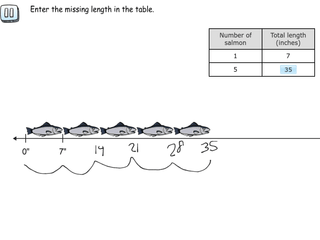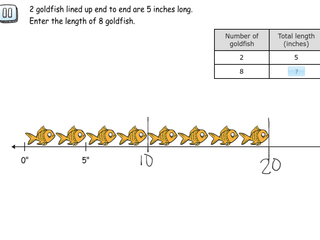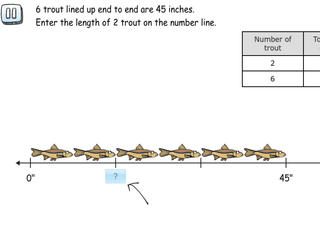# Interactive Problem Bank

•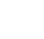Fraction Circles
•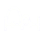Number Lines
•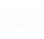Fraction Bars
•Sets
•Area Models
•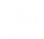Base Ten Blocks

## Making Sense of Ratios

• Students understand the concept of ratios.
• Students use visual models including paint mixers object measurments to explore ratios.
• Students use ratio and proportional reasoning to solve real-world and mathematical problem.
• Students understand the use of mathematical language to describe a relationship between two quantities.

### Ratio Tables and the Number Line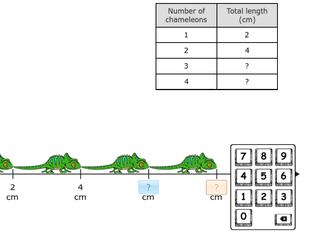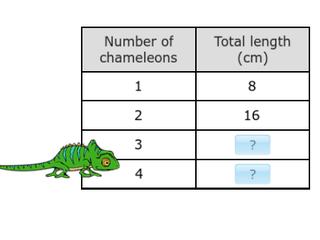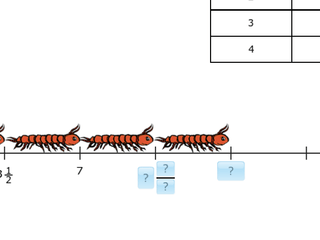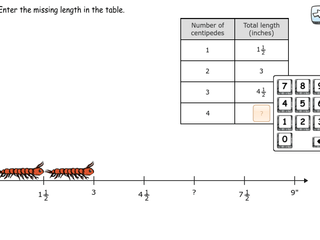### Finding Lengths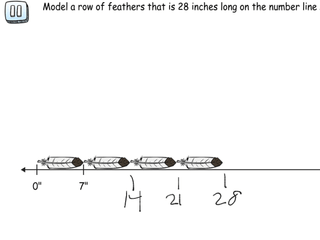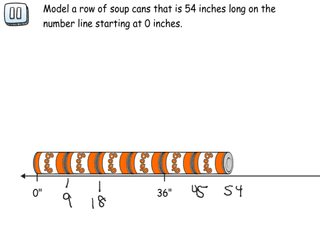### Scaling Lengths